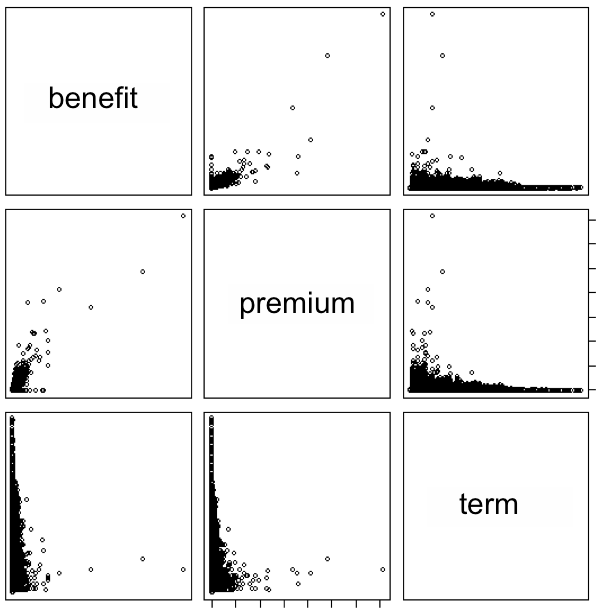# Cluster Modelling for Insurance Portfolios

## Abstract

Recently I came across an article by Milliman (an actuarial and consulting firm) called Cluster Modelling: A practical and robust approach for achieving high improvements in model run-times for SST and Solvency II. Here I will analyse their approach and point out a disadvantage in connection with reasonable large protfolios.

## Introduction

Millinan's article is about compressing huge insurance portfolios so that a stochastic projection using an actuarial model system software (like Prophet, Moses etc.) becomes practical in terms of run time. This procedure is also sometimes called model point compression or inforce data compression.

First of all I found the article quite readable, among others because Milliman clearly stated their algorithmic approach and did not obfuscate their approach by vague and obscure formulations. I also very much agree with their analysis that model point creation using clustering techniques (together with a sound validation scheme) outperformance traditional model point grouping techniques.Projection of an insurance protfolio along three main dimensions drawn as a scatter plot.

Nevertheless I think their approach has at least one drawback, which makes it difficult to apply to bigger portfolios.

## Algorithm

In principal Milliman applies a so called agglomerative hierarchical clustering algorithm. Their approach differs in so far that they stop the algorithm when the desired number of clusters -- i.e. model points -- is reached (the classical agglomerative hierarchical clustering would continue until a single cluster containing all data points is formed). From "Step 6" on page 2 it seems Milliman applies the so called single-linkage criterium to calculate the distances between clusters. The distance between cluster $X$ and $Y$ is given by the distance of their nearest points,

$$D(X,Y)=\min_{x\in X, y\in Y}d(x,y)$$

where $d(x,y)$ is the Euclidean distance.

## Analysis

A hierarchical clustering algorithm has a run time and memory complexity of $\mathcal{O}(n^2)$. In practical terms, you have to construct the so called dissimilarity matrix

$$\mathbf{D}=\left( \begin{array}{cccc} 0 & d_{12} & d_{13} & \ldots \\ d_{21} & 0 & d_{23} & \ldots \\ d_{31} & d_{32} & 0 & \ldots\\ \vdots & \vdots & \vdots & \ddots \end{array} \right),$$

where $d_{ij}=d(x_i,x_j)$. Since $\mathbf{D}$ is symmetric we only have to calculate (and keep in memory) $n(n-1)/2$ distances. Using the dissimilarity matrix we can implement the single linkage hierarchical clustering. Without going into details, one approach would be to search for the nearest neighbor in the matrix. When merging two clusters we have to delete one column and update the other so that it represents the distances from the newly formed cluster. It is possible to do this in a way so that the run time complexity does not increase above $\mathcal{O}(n^2).$

Let us focus on the dissimilarity matrix and its memory footprint. Assuming we encode each distance as a 4 byte C/C++ float type we can calculate following examples (the first corresponds to Milliman's example):

portfolio size no calculations memory in GB
$210,000$ $22.05\cdot 10^9$ $82.1$
$500,000$ $125.00\cdot 10^9$ $465.7$
$1,000,000$ $500.00\cdot 10^9$ $1,862.6$
$2,000,000$ $2,000.00\cdot 10^9$ $7,450.6$

Under certain circumstances it could be reasonable to use separate runs for each segment (line of business) and so lower the memory and run time complexity to the square of the maximum segment size. Also you could start with a reasonable sized sample of the original portfolio (say you work with a sample of 200,000 instead of your original 2 million). Still the $\mathcal{O}(n^2)$ run time and memory complexity stays as a very unpleasant constraint.

## Summary

Of cause the hierarchical approach taken by Milliman has it's advantages like being conceptional very simply or being deterministic (there is no random component in the algorithm, as with most partitioning approaches like k-means). My point is, you shouldn't use a $\mathcal{O}(n^2)$ clustering algorithm at all. Or do you really want to buy and manage a terra byte server farm? From my experience (I successfully introduced a k-means based approach with several insurance companies each having far more than 1 million policies) a k-means variant does the same job but with $\mathcal{O}(n)$ memory and $\mathcal{O}(n\cdot k)$ run time complexity (where $k\ll n$ is the number of iterations) which drastically decreases hardware costs and run time.